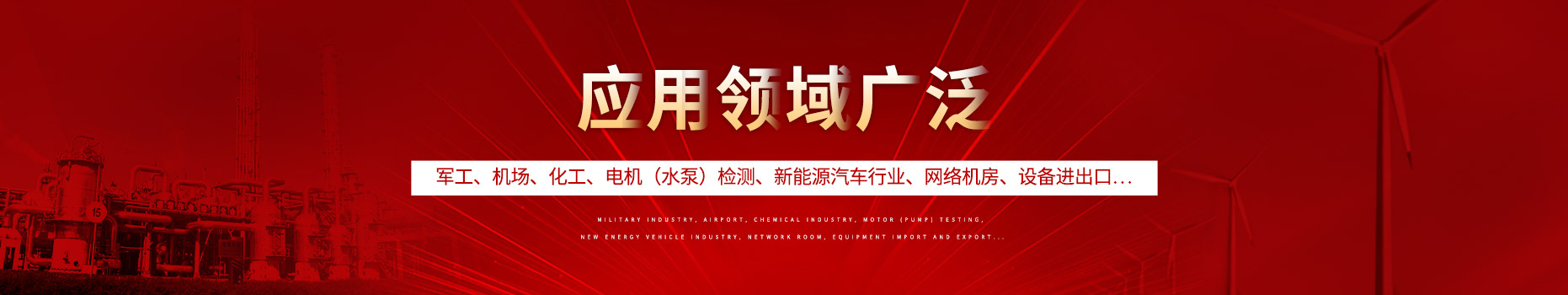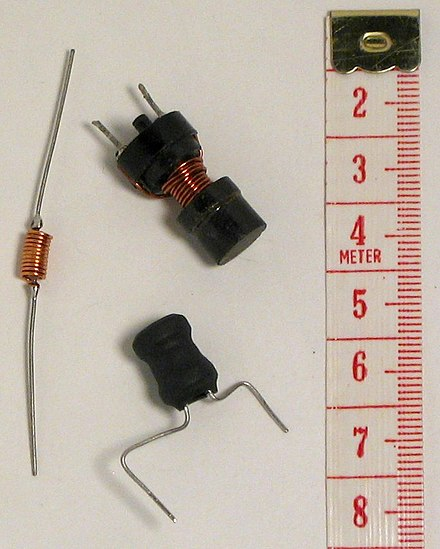400-088-6921155-8888-6921#### 产品分类# 电感器件

电感（英语：inductor）是一种电路器件，会因为通过的电流的改变而产生电动势，从而抵抗电流的改变。这属性称为电感。

电感器一词，通常只用来称呼以自感或其效应为主要工作情况的器件。非以自感为主的，习惯上大多称呼它的其他名称，平常不以电感器称呼，例如：变压器、马达里的电磁线圈绕组等。

在中文里，电感器一词在口语上也会被简称为电感，但如需严谨表达为实体物件的情况，仍宜称为电感器。

## 概述

通俗地说，穿过一个闭合导体回路的磁感线条数称为磁通量。由于穿过闭合载流导体（很多情况是线圈）的磁场在其内部形成的磁通量变化，根据法拉第电磁感应定律，闭合导体将产生一个电动势以“反抗”这种变化，即电磁感应现象。电感器件的电磁感应分为自感应和互感应，自身磁场在线圈内产生磁通量变化导致的电磁感应现象，称为“自感应”现象；外部磁场在线圈里磁通量变化产生的电磁感应现象，称为“互感应”现象。

比如，当电流以1安培/秒的变化速率穿过一个1亨利的电感器件，则引起1伏特的感应电动势。当缠绕导体的导线匝数增多，导体的电感也会变大，不仅匝数，每匝（环路）面积，连缠绕材料都会影响电感大小。此外，用高渗透性材料缠绕导体也会令磁通量增加。

电感器件即利用这种感应的原理，在电路中发挥了许多作用。

## 储存的能量

一个电感器件储存的能量（单位：焦耳）等于流经它的电流建立磁场所做的功，其值由下式给出：

{\displaystyle E_{\mathrm{stored}}={1\over 2}LI^{2}}E_{{\mathrm{stored}}}={1\over 2}LI^{2}

其中L为电感，I为流经电感的电流。

上述的关系仅适用在电流和磁通呈线性，尚未进入磁饱和的电感器件。

若针对电感器件，要计算在时间{\displaystyle t_{0}}t_{0}到{\displaystyle t_{1}}t_{1}之间，电感器件可以储存的能量，可以用下式计算：

{\displaystyle E=\int _{t_{0}}^{t_{1}}\!P(t)\,dt={\frac{1}{2}}LI(t_{1})^{2}-{\frac{1}{2}}LI(t_{0})^{2}}E=\int _{{t_{0}}}^{{t_{1}}}\!P(t)\,dt={\frac{1}{2}}LI(t_{1})^{2}-{\frac{1}{2}}LI(t_{0})^{2}

## 水压模型

电流可以被模拟为水流一样，电感器件相当于被水流驱动的涡轮中的“飞轮”。电压与电流改变的量成正比，所以电流的急速改变会产生强力的电压。相似地，流向涡轮的水流被突然干扰时会产生巨大的压力。于变压器中的磁力交流没有被有效地以模型形式模拟出来。

## 电感器件结构电感可由电导材料盘绕磁芯制成，典型的如铜线，也可把磁芯去掉或者用铁磁性材料代替。比空气的磁导率高的芯材料可以把磁场更紧密的约束在电感器件周围，因而增大了电感。电感有很多种，大多以外层瓷釉线圈（enamel coated wire）环绕铁素体线轴制成，而有些防护电感把线圈完全置于铁素体内。一些电感器件的芯可以调节。由此可以改变电感大小。小电感能直接蚀刻在印刷电路板上，用一种铺设螺旋轨迹的方法。小值电感也可用以制造晶体管同样的工艺制造在集成电路中。在这些应用中，铝互连线被经常用做传导材料。不管用何种方法，基于实际的约束应用最多的还是一种叫做“旋转子”的电路，它用一个电容和主动器件表现出与电感器件相同的特性。用于隔高频的电感器件经常用一根穿过磁柱或磁珠的金属丝构成。

## 在电子电路中

像电容器件反抗电压的变化一样，电感器件反抗电流的变化。一个理想电感器件应对直流电不呈电阻性，然而只有超导电感器件才会产生零电阻。

一般来说，随时间变化的电压v(t)与随时间变化的电流i(t)在一个电感为L的电感器件上呈现的关系可以用微分方程来表示：

{\displaystyle v(t)=L{\frac{di(t)}{dt}}}{\displaystyle v(t)=L{\frac{di(t)}{dt}}}。

当有正弦交流电穿过电感器件时，会产生正弦电压。电压的幅度与电流的幅度（{\displaystyle I_{P}}I_{P}）与电流的频率（f）的乘积成比例。

{\displaystyle i(t)=I_{P}\sin(2\pi ft)\,}i(t)=I_{P}\sin(2\pi ft)\,

{\displaystyle{\frac{di(t)}{dt}}=2\pi fI_{P}\cos(2\pi ft)}{\frac{di(t)}{dt}}=2\pi fI_{P}\cos(2\pi ft)

{\displaystyle v(t)=2\pi fLI_{P}\cos(2\pi ft)\,}v(t)=2\pi fLI_{P}\cos(2\pi ft)\,

在这种情况下，电流与电压的相位相差90度，（电流落后电压）

当于电路分析中使用拉普拉斯变换，一个没有初始电流的理想电感器件的阻抗能于s域被表述成：

{\displaystyle Z(s)=sL\,}Z(s)=sL\,

L为电感

s为复频率

如果电感器件没有起始电流，那它可以被表述成：

附加一个电压来源，以串联形式与电感器件连接着，电压来源的值为：

{\displaystyle LI_{0}\,}LI_{0}\,

(请留意电压来源应该有与初始电流相反的极性）

或是附加一个电流来源，以并联形式与电感器件连接着，电流来源的值为：

{\displaystyle{\frac{I_{0}}{s}}}{\frac{I_{0}}{s}}

L为电感

{\displaystyle I_{0}}I_0为电感器件的初始电流

主条目：串联与并联电路

并联电路中的电感器件每个都有相同的电势差。其总的等效电感（Leq）：

并联电感器件

{\displaystyle{\frac{1}{L_{\mathrm{eq}}}}={\frac{1}{L_{1}}}+{\frac{1}{L_{2}}}+\cdots+{\frac{1}{L_{n}}}}{\frac{1}{L_{{\mathrm{eq}}}}}={\frac{1}{L_{1}}}+{\frac{1}{L_{2}}}+\cdots+{\frac{1}{L_{n}}}

通过串联电感的电流保持不变，但每个电感器件上的电压可不同。其电压之和等于总电压。总电感：

串联电感器件，其所经电流相同

{\displaystyle L_{\mathrm{eq}}=L_{1}+L_{2}+\cdots+L_{n}\,\!}L_{{\mathrm{eq}}}=L_{1}+L_{2}+\cdots+L_{n}\,\!

这种简单的关系只有在没有磁场互耦（mutual coupling）的条件下才成立。

## 品质因数Q

一个理想的电感器件是不会因流经线圈的电流的大小而改变其敏感度。但是于实际环境下，线圈内的金属线会令电感器件带有绕组电阻。由于绕组电阻是以串联著电感器件的电阻形式出现，所以亦被称为串联电阻。由于串联电阻的存在，实际电感器件的特性会不同于理想电感，可以用品质因数表示电感和电阻之的比例。

一个电感器件的品质因数（简称Q）是它处于某一特定频率时，它的电感电抗和电阻之间的比例，这个比例是用来量度电感器件的有效程度。品质因数越高，电感器件的表现越相似现想中电感器件的表现。

电感器件的品质因数Q能由以下方程序可得，R是电感器件的内部电抗：

{\displaystyle Q={\frac{\omega{}L}{R}}}Q={\frac{\omega{}L}{R}}

使用铁磁性材料而其他部分不变的话，电感会上升，因此品质因数会被提高。但是若频率上升时，铁磁性材料的电感会降低，也就是电感是频率的变数。所以于甚高频（VHF）或更高频的情况下，会倾向使用空气核心。使用铁磁性核心的电感器件可能会于大量电流流入时进入饱和状态，引致电感及品质因数下降。使用空气核心能避免这种现象。一个经良好设计的含空气核心的电感器件能有高达几百的品质因数。

一个近乎理想的电感器件（即近乎无限的的品质因数）可以由以下方法所制：将由超导合金所制的线圈浸入液态氦或液态氮中。这会令电线处于极低温状态，而绕组电阻会消失。因为超导电感器件的效能极近乎理想中的电感器件，它可以储存大量电能于磁场内。（见超导储能）

相同条件下内阻越大，品质因数越小。品质因数可以看做是衡量电感器件好坏的标准之一，品质因数越高通常意味着电感的品质越好。

## 公式

以下的表列出一些简单形状电感器，其电感量近似公式。

架构公式注解

无铁心的圆柱形电感{\displaystyle L={\frac{\mu _{0}KN^{2}A}{l}}}{\displaystyle L={\frac{\mu _{0}KN^{2}A}{l}}}

L=电感单位亨利（H）

μ0=自由空间的磁导率=4{\displaystyle\pi}\pi×10-7 H/m

K=Nagaoka系数

N=匝数

A=环绕的横断面积，单位：平方米（m2）

l=盘绕长度，单位：米（m）

Nagaoka系数（K）的计算十分复杂，通常需要查表得到。但一般可取近似值{\displaystyle K\approx 1}{\displaystyle K\approx 1}（适用于使用细导线紧密排绕，而且线圈长度远大于线圈直径的单层电感）

线形导体{\displaystyle L={\frac{\mu _{0}}{2\pi}}\left(l\ln\left[{\frac{1}{c}}\left(l+{\sqrt{l^{2}+c^{2}}}\right)\right]-{\sqrt{l^{2}+c^{2}}}+c+{\frac{l}{4+c{\sqrt{{\frac{2}{\rho}}\omega\mu}}}}\right)}L={\frac{\mu _{0}}{2\pi}}\left(l\ln\left[{\frac{1}{c}}\left(l+{\sqrt{l^{2}+c^{2}}}\right)\right]-{\sqrt{l^{2}+c^{2}}}+c+{\frac{l}{4+c{\sqrt{{\frac{2}{\rho}}\omega\mu}}}}\right)

L=电感

l=导线长度

c=导线半径

μ0=自由空间的磁导率=4{\displaystyle\pi}\pi×10−7 H/m

μ=导体相对导磁率

p=电阻

ω=相频率

在ω=0或ω=∞时是准确的

{\displaystyle L={\frac{1}{5}}l\left[\ln\left({\frac{4l}{d}}\right)-1\right]}L={\frac{1}{5}}l\left[\ln\left({\frac{4l}{d}}\right)-1\right]

L=电感（nH）

l=导线长度（mm）

d=导线直径（mm）

f=频率

Cu or Al（铜或是铝，相对导磁率接近1）

l&gt;100 d

d2 f&gt;1 mm2 MHz

{\displaystyle L={\frac{1}{5}}l\left[\ln\left({\frac{4l}{d}}\right)-{\frac{3}{4}}\right]}L={\frac{1}{5}}l\left[\ln\left({\frac{4l}{d}}\right)-{\frac{3}{4}}\right]

L=电感（nH）

l=导线长度（mm）

d=导线直径（mm）

f=频率

Cu or Al（铜或是铝，相对导磁率接近1）

l&gt;100 d

d2 f&lt;1 mm2 MHz

3.短圆柱盘绕无芯（空气）电感器件的电感：

{\displaystyle L={\frac{r^{2}N^{2}}{9r+10l}}}L={\frac{r^{2}N^{2}}{9r+10l}}

L=电感单位µH

r=缠绕的外环半径单位英寸

l=缠绕长度单位英寸

N=匝数

4.多层空气芯电感器件：

{\displaystyle L={\frac{0.8r^{2}N^{2}}{6r+9l+10d}}}L={\frac{0.8r^{2}N^{2}}{6r+9l+10d}}

L=电感单位µH

r=缠绕平均半径单位英寸

l=绕线物理长度单位英寸

N=匝数

d=缠绕深度单位英寸（即，外半径减去内半径）

5.平螺旋型空芯电感：

{\displaystyle L={\frac{r^{2}N^{2}}{(2r+2.8d)\times 10^{5}}}}L={\frac{r^{2}N^{2}}{(2r+2.8d)\times 10^{5}}}

L=电感单位H

r=缠绕平均半径单位米

N=匝数

d=缠绕深度单位米（即，外半径减去内半径）

因此一个8匝的螺旋型盘绕，平均半径25mm，深度10mm的电感器件，电感为5.13µH。

同样的公式改用英制单位：

{\displaystyle L={\frac{r^{2}N^{2}}{8r+11d}}}L={\frac{r^{2}N^{2}}{8r+11d}}

L=电感单位µH

r=缠绕平均半径单位英寸

N=匝数

d=缠绕深度单位英寸（即，外半径减去内半径）

6.环形铁心的绕阻电感（核心物料的的圆形横切面的相对导率为{\displaystyle\mu _{r}}\mu _{r}）

{\displaystyle L=\mu _{0}\mu _{r}{\frac{N^{2}r^{2}}{D}}}L=\mu _{0}\mu _{r}{\frac{N^{2}r^{2}}{D}}

L=电感单位H

μ0=真空磁导率=4{\displaystyle\pi}\pi×10-7 H/m

μr=核心物料的相对导率

N=匝数

r=缠绕平均半径单位米

D=环形线圈的总直径单位米

## 应用

• 　　电感器件与电容器件及其他一些器件结合可以形成调谐电路，可以放大或过滤一些特定的信号频率。

• 　　大电感可用于电源的阀门（chokes），以前也经常与滤波器联用用于去除直流输出的冗余和波动成分。

• 　　磁珠或环绕电缆可产生小电感可阻止传输线中的射频干扰。

• 　　小的电容/电感还可结合产生调谐电路用于无线电的收发。

• 　　两个或多个电感器件之间有耦合磁通量可形成变压器，变压器是电力电源系统的基本组件。变压器的效率随着频率的增加而减小，但高频变压器的体积也变的很小，这也是为什么一些飞行器用400赫兹交流电而不是通常的50或60赫兹，用小型变压器而节省了大量的载重。

• 　　在开关式电源中，电感器件被做为储能器件。电感器件随着调整器的转换频率的特定部分而储能，而在周期后半部分释放能量。其能量转换比决定了输入输出电压比。这个XL用于补充主动半导体设备可用来精确控制电压。

• 　　电感器件也被应用于电力传输系统，用来降低系统电压或限制疵电流，这些通常被用于反应堆。相比其他器件电感器件要显得大而重，所以在现代设备里以减少了其应用；有些固态开关电源去掉了大变压器，电路转为使用小的电感器件，有些则由回转器电路模拟。

### 相关新闻

##### 联系方式

15588886921

400热线

400-0886921

2466458158@qq.com

##### 二维码